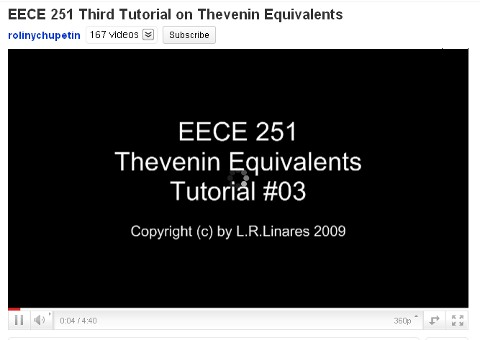# Third Tutorial on Thevenin EquivalentThis is Thevenin equivalents video tutorial part 3. This video shows how to calculate Thevenin equivalents with control source and an independent source. IN this video, the Thevenin voltage between p and q is calculated using open circuit test. In this video, circuit maker is used to calculate the Voc. When the circuit running, Voc is obtained about 4.179V. To obtain the current, this video uses short circuit method. the current of circuit is 3A. Thevenin voltage of the circuit is 4.179V. R Thevenin is calculated by dividing the Voc with Isc.